• 一维卷积神经网络的理解
千次阅读
2020-11-07 20:58:03

设输入的数据维度是B x S x T

一维卷积神经网络在维度S上进行卷积

如下，设置一维卷积网络的输入通道为16维，输出通道为33维，卷积核大小为3，步长为2

# in_channels: 16
# out_channels: 33
# kernel_size: 3
m = nn.Conv1d(16, 33, 3, stride=2)
input = torch.randn(20, 16, 50)
output = m(input)
# shape of output is ([20, 33, 24])
# 最后一个维度:
# (50 - 3)/2 + 1 = 24
print(output.shape)

如上所述，输入维度为20 x 16 x 50

经过一维卷积后输出维度是20 x 33 x 24

第二个维度从16变为33，因为一维卷积输入通道为16，对应输入的第二个维度，一维卷积输出为33，对应输出的第二个维度

最后一个维度从50变为24，将参数带入公式[(n+2p-f) / s + 1]向下取整得到[(50-3)/2 + 1] = 24

而全连接神经网络对维度T进行卷积

使用和上述相同的输入维度，设置全连接神经网络的输入维度为input的最后一个维度50，输出维度为33

m1 = nn.Linear(50, 33)
output1 = m1(input)
# shape of output1 is ([20, 16, 33])
print(output1.shape)


将输入通过全连接神经网络后得到输出维度为20 x 16  x 33

即，全连接神经网络只在输入的最后一个维度进行卷积

更多相关内容
• ## 【keras】一维卷积神经网络多分类

万次阅读 多人点赞 2020-06-20 13:05:05
刚刚接触到深度学习，前2个月的时间里，我用一维卷积神经网络实现了对于一维数据集的分类和回归。由于在做这次课题之前，我对深度学习基本上没有过接触，所以期间走了很多弯路。 在刚刚收到题目的要求时，我选择...

刚刚接触到深度学习，前2个月的时间里，我用一维的卷积神经网络实现了对于一维数据集的分类和回归。由于在做这次课题之前，我对深度学习基本上没有过接触，所以期间走了很多弯路。

在刚刚收到题目的要求时，我选择使用TensorFlow来直接编写函数，结果由于没有什么基础，写了一个周我就放弃了，改用keras来完成我的任务。

用keras来搭建神经网络其实很简单。我把自己的网络模型和数据集分享给大家，一起交流一起进步。

我们要完成的任务是对一些给定的湿度特征数据进行分类，多分类就是最简单最入门的深度学习案例。

我们要完成分类任务的数据集，247*900大小的一个矩阵，其中包含900条湿度信息，每条湿度信息由246位比特构成，最后一位表示湿度的类别，数值1-9，也就是每种湿度有100条。大家可以点击下边的链接自取。

数据集-用做分类.csv

或者百度网盘：

链接：https://pan.baidu.com/s/1R0Ok5lB_RaI2cVHychZuxQ
提取码：9nwi
复制这段内容后打开百度网盘手机App，操作更方便哦

首先是数据的导入：

# 载入数据
X = np.expand_dims(df.values[:, 0:246].astype(float), axis=2)
Y = df.values[:, 246]

X是900条数据的特征信息，Y表示真实类别。特别注意：这里的X在读取的时候矩阵增加了一维。使用一维卷积神经网络Conv1D的时候必须要事先这样对数据集进行处理，而只使用深度网络Dense时不需要额外的增加一维。

具体细节大家可以看我之前写过的一篇博客，比较细的区分了这两种情况：

Conv1D层与Dense层的连接

没有接触过的读者可以查一下只使用深度层和使用卷积层的区别。CNN眼里只有局部小特征，而DNN只有全局大特征。

最普通的深层神经网络包含多层神经元，从输入信号中提取信息。每个神经元接受来自前一层神经元的输入，并通过权重和非线性将它们组合起来。与普通神经网络中每个神经元与前一层的所有神经元连接不同，CNN中的每个神经元只与前一层的少数神经元局部连接。而且，CNN同一层的所有神经元都有相同的权重。CNN层中神经元的值通常被称为特征映射（features maps），因为它们可以被看作是对应于输入的不同部分的特征。卷积神经网络可以很好地捕获出原数据中的局部简单特征，随着层数的增加，产生的特征映射会捕获输入信号越来越多的全局和更复杂的属性。它可以在一条数据中较短的片段中获得感兴趣的特征，并且该特征在该数据片段中的位置不具有高度相关性

紧接着是对湿度类别的编码：

# 湿度分类编码为数字
encoder = LabelEncoder()
Y_encoded = encoder.fit_transform(Y)
Y_onehot = np_utils.to_categorical(Y_encoded)

Y_onehot编码是为了让程序自己来区分每一条特征信息而添加的编码。一个简单的例子解释一下：

这一步转化工作我们可以利用keras中的np_utils.to_categorical函数来进行。

“one-hot编码。one-hot编码的定义是用N位状态寄存器来对N个状态进行编码。比如[0,...,0]，[1,...,1]，[2,...,2]有3个分类值,因此N为3,对应的one-hot编码可以表示为100,010,001。”

我们将原始的数据集划分为训练集和测试集：

# 划分训练集，测试集
X_train, X_test, Y_train, Y_test = train_test_split(X, Y_onehot, test_size=0.3, random_state=0)

我这里把原始数据集以7：3的比例划分为训练集和测试集。训练集用来逼近真实类别，测试集来测试效果。

接着的是网络模型的搭建：

# 定义神经网络
def baseline_model():
model = Sequential()
plot_model(model, to_file='./model_classifier.png', show_shapes=True) # 保存网络结构为图片，这一步可以直接去掉
print(model.summary()) # 显示网络结构
return model

这一步往往是最核心的一步，网络模型通过卷积层来提取特征，在分类任务中，网络的最后一层为每个类。分类算法得到的是一个决策面，通过对比湿度信息属于每一类湿度的概率，进而对号入座。

经过多次调参尝试，最后我使用7层Conv1D来提取特征值，每两层Conv1D后添加一层MaxPooling1D来保留主要特征，减少计算量。每层卷积层使用双曲正切函数tanh（hyperbolic tangent function）来提高神经网络对模型的表达能力。tanh经常被运用到多分类任务中用做激活函数。

神经网络本就具有不可解释性，一般卷积核和全连接的结点数按照2的指数次幂来取。

Flatten()层作为中间层来链接卷积神经网络和全连接层。

神经网络被训练来最小化一个损失函数（Softmax loss），该函数捕捉到网络的特征输出与给定的期望输出之间的差异。9个输出结点对应九个类别的湿度，Softmax将每个特征数据匹配到概率最大的特征类别。

交叉熵损失函数（categorical crossentropy）作为模型训练的损失函数，它刻画的是当前学习到的概率分布与实际概率分布的距离，也就是损失函数越小，两个概率分布越相似，此时损失函数接近于0。

我将网络模型绘制成了AlexNet风格的示意图：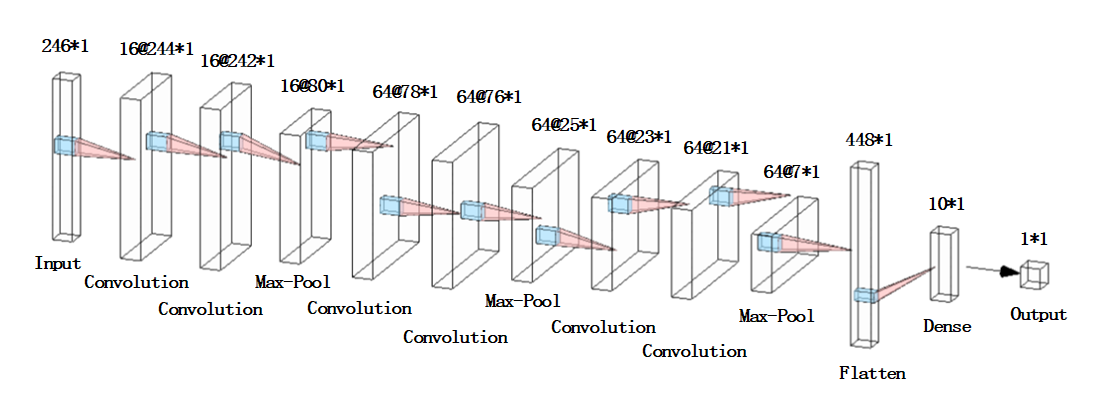这里也给大家安利下这个很好用的绘制神经网络的工具：

http://alexlenail.me/NN-SVG/AlexNet.html

搭建好网络之后就可以开始训练了。

# 训练分类器
estimator = KerasClassifier(build_fn=baseline_model, epochs=40, batch_size=1, verbose=1)
estimator.fit(X_train, Y_train)

batch_size设为1，然后一共训练40期。

这些数据大家都可以根据自己的实际情况做出调整和优化。

到这一步已经是搭建和训练的部分全部结束了。

紧接着是测试集来验证训练的准确性。

为了每次测试前不重复训练，我们将训练的模型保存下来：

# 将其模型转换为json
model_json = estimator.model.to_json()
with open(r"C:\Users\Desktop\model.json",'w')as json_file:
json_file.write(model_json)# 权重不在json中,只保存网络结构
estimator.model.save_weights('model.h5')

下面是读取模型的部分，这一部分完全可以写到另一个函数里：

# 加载模型用做预测
json_file = open(r"C:\Users\Desktop\model.json", "r")
json_file.close()
# 分类准确率
print("The accuracy of the classification model:")
print('%s: %.2f%%' % (loaded_model.metrics_names, scores * 100))
# 输出预测类别
print("predicted label:\n " + str(predicted_label))

当然我们也希望可以直观的显示出模型的分类精度，和训练的过程。

混淆矩阵经常用来表示分类的效果。

#显示混淆矩阵
plot_confuse(estimator.model, X_test, Y_test)

当然也需要我们自己写出来：

# 混淆矩阵定义
def plot_confusion_matrix(cm, classes,title='Confusion matrix',cmap=plt.cm.jet):
cm = cm.astype('float') / cm.sum(axis=1)[:, np.newaxis]
plt.imshow(cm, interpolation='nearest', cmap=cmap)
plt.rcParams['font.sans-serif'] = ['SimHei']  # 用来正常显示中文标签
plt.rcParams['axes.unicode_minus'] = False  # 用来正常显示负号
plt.colorbar()
tick_marks = np.arange(len(classes))
plt.xticks(tick_marks,('0%','3%','5%','8%','10%','12%','15%','18%','20%','25%'))
plt.yticks(tick_marks,('0%','3%','5%','8%','10%','12%','15%','18%','20%','25%'))
thresh = cm.max() / 2.
for i, j in itertools.product(range(cm.shape), range(cm.shape)):
plt.text(j, i, '{:.2f}'.format(cm[i, j]), horizontalalignment="center",color="white" if cm[i, j] > thresh else "black")
plt.tight_layout()
plt.ylabel('真实类别')
plt.xlabel('预测类别')
plt.savefig('test_xx.png', dpi=200, bbox_inches='tight', transparent=False)
plt.show()

# 显示混淆矩阵
def plot_confuse(model, x_val, y_val):
predictions = model.predict_classes(x_val)
truelabel = y_val.argmax(axis=-1)   # 将one-hot转化为label
conf_mat = confusion_matrix(y_true=truelabel, y_pred=predictions)
plt.figure()
plot_confusion_matrix(conf_mat, range(np.max(truelabel)+1))


如果好奇的话你也可以看看每层卷积层到底学到了什么：

# 可视化卷积层
visual(estimator.model, X_train, 1)

但是我觉得看一维卷积层的特征没啥意义，毕竟都是一些点，看起来有点非人类。

# 卷积网络可视化
def visual(model, data, num_layer=1):
# data:图像array数据
# layer:第n层的输出
layer = keras.backend.function([model.layers.input], [model.layers[num_layer].output])
f1 = layer([data])
print(f1.shape)
num = f1.shape[-1]
print(num)
plt.figure(figsize=(8, 8))
for i in range(num):
plt.subplot(np.ceil(np.sqrt(num)), np.ceil(np.sqrt(num)), i+1)
plt.imshow(f1[:, :, i] * 255, cmap='gray')
plt.axis('off')
plt.show()

最后运行的所有结果如下：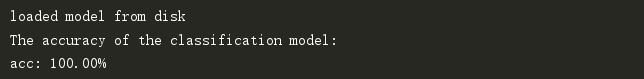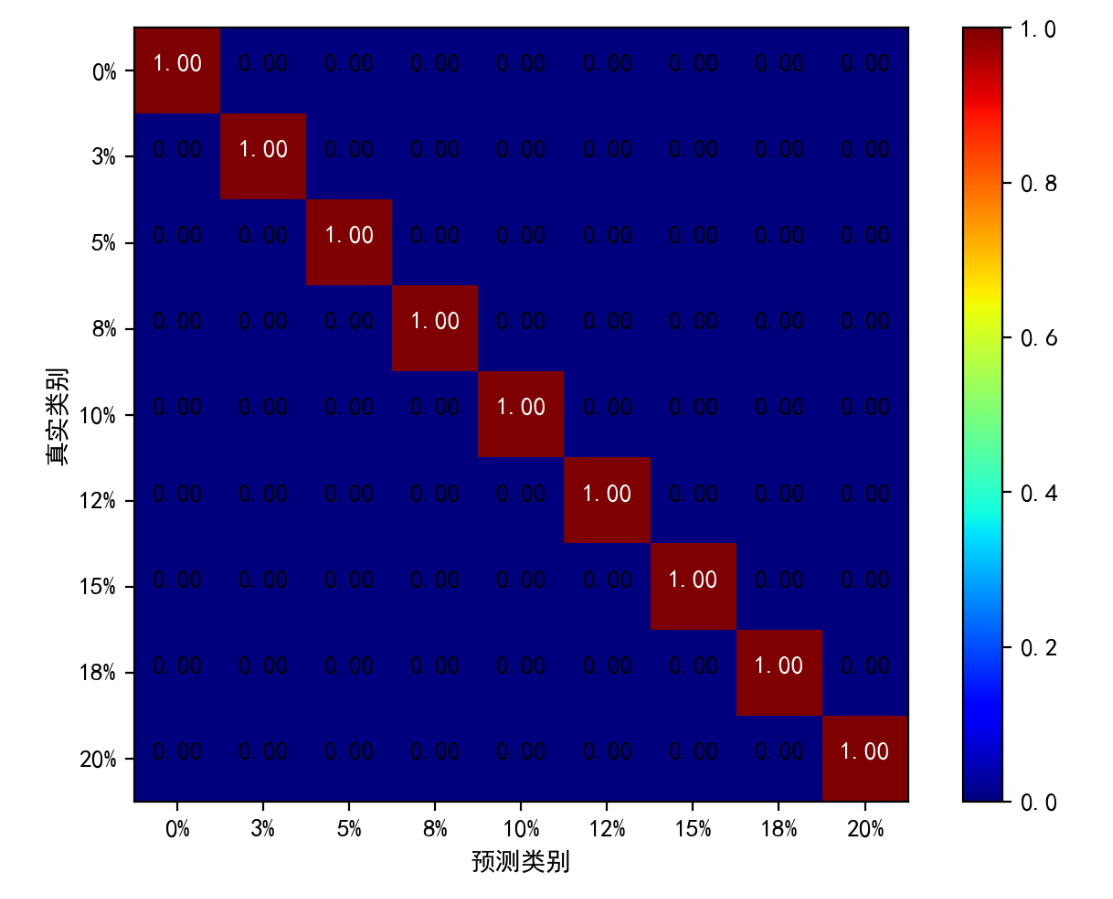这个结果这么好纯粹是因为数据比较好，分类的准确率很大程度都是依赖你数据集本身的区分度的。

下面是数据集的特征图，从图上就可以看出数据的层次性很直观。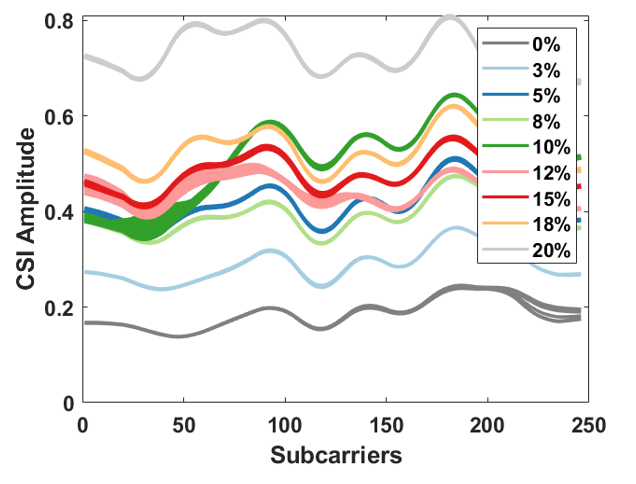当然我给大家上传的这部分数据是我这边结果最好的一批，其他数据集准确度达不到这么高。

下面贴出整个分类过程的完整代码：

# -*- coding: utf8 -*-
import numpy as np
import pandas as pd
import keras
from keras.models import Sequential
from keras.wrappers.scikit_learn import KerasClassifier
from keras.utils import np_utils,plot_model
from sklearn.model_selection import cross_val_score,train_test_split,KFold
from sklearn.preprocessing import LabelEncoder
from keras.layers import Dense,Dropout,Flatten,Conv1D,MaxPooling1D
from keras.models import model_from_json
import matplotlib.pyplot as plt
from sklearn.metrics import confusion_matrix
import itertools

# 载入数据
X = np.expand_dims(df.values[:, 0:246].astype(float), axis=2)
Y = df.values[:, 246]

# 湿度分类编码为数字
encoder = LabelEncoder()
Y_encoded = encoder.fit_transform(Y)
Y_onehot = np_utils.to_categorical(Y_encoded)

# 划分训练集，测试集
X_train, X_test, Y_train, Y_test = train_test_split(X, Y_onehot, test_size=0.3, random_state=0)

# 定义神经网络
def baseline_model():
model = Sequential()
plot_model(model, to_file='./model_classifier.png', show_shapes=True)
print(model.summary())
return model

# 训练分类器
estimator = KerasClassifier(build_fn=baseline_model, epochs=40, batch_size=1, verbose=1)
estimator.fit(X_train, Y_train)

# 卷积网络可视化
# def visual(model, data, num_layer=1):
#     # data:图像array数据
#     # layer:第n层的输出
#     layer = keras.backend.function([model.layers.input], [model.layers[num_layer].output])
#     f1 = layer([data])
#     print(f1.shape)
#     num = f1.shape[-1]
#     print(num)
#     plt.figure(figsize=(8, 8))
#     for i in range(num):
#         plt.subplot(np.ceil(np.sqrt(num)), np.ceil(np.sqrt(num)), i+1)
#         plt.imshow(f1[:, :, i] * 255, cmap='gray')
#         plt.axis('off')
#     plt.show()

# 混淆矩阵定义
def plot_confusion_matrix(cm, classes,title='Confusion matrix',cmap=plt.cm.jet):
cm = cm.astype('float') / cm.sum(axis=1)[:, np.newaxis]
plt.imshow(cm, interpolation='nearest', cmap=cmap)
plt.rcParams['font.sans-serif'] = ['SimHei']  # 用来正常显示中文标签
plt.rcParams['axes.unicode_minus'] = False  # 用来正常显示负号
plt.colorbar()
tick_marks = np.arange(len(classes))
plt.xticks(tick_marks,('0%','3%','5%','8%','10%','12%','15%','18%','20%','25%'))
plt.yticks(tick_marks,('0%','3%','5%','8%','10%','12%','15%','18%','20%','25%'))
thresh = cm.max() / 2.
for i, j in itertools.product(range(cm.shape), range(cm.shape)):
plt.text(j, i, '{:.2f}'.format(cm[i, j]), horizontalalignment="center",color="white" if cm[i, j] > thresh else "black")
plt.tight_layout()
plt.ylabel('真实类别')
plt.xlabel('预测类别')
plt.savefig('test_xx.png', dpi=200, bbox_inches='tight', transparent=False)
plt.show()

# seed = 42
# np.random.seed(seed)
# kfold = KFold(n_splits=10, shuffle=True, random_state=seed)
# result = cross_val_score(estimator, X, Y_onehot, cv=kfold)
# print("Accuracy of cross validation, mean %.2f, std %.2f\n" % (result.mean(), result.std()))

# 显示混淆矩阵
def plot_confuse(model, x_val, y_val):
predictions = model.predict_classes(x_val)
truelabel = y_val.argmax(axis=-1)   # 将one-hot转化为label
conf_mat = confusion_matrix(y_true=truelabel, y_pred=predictions)
plt.figure()
plot_confusion_matrix(conf_mat, range(np.max(truelabel)+1))

# 将其模型转换为json
model_json = estimator.model.to_json()
with open(r"C:\Users\316CJW\Desktop\毕设代码\model.json",'w')as json_file:
json_file.write(model_json)# 权重不在json中,只保存网络结构
estimator.model.save_weights('model.h5')

# 加载模型用做预测
json_file = open(r"C:\Users\Desktop\model.json", "r")
json_file.close()
# 分类准确率
print("The accuracy of the classification model:")
print('%s: %.2f%%' % (loaded_model.metrics_names, scores * 100))
# 输出预测类别
print("predicted label:\n " + str(predicted_label))
#显示混淆矩阵
plot_confuse(estimator.model, X_test, Y_test)

# 可视化卷积层
# visual(estimator.model, X_train, 1)

但是，湿度值到底是连续的数值，分类任务验证了我们数据集特征的可分辨性。

下一篇博客中，我将对数据集稍作修改，将湿度类别改为真实湿度值。

利用卷积神经网络来提取特征，实现线性回归，二者同出一脉。

【keras】一维卷积神经网络做回归

比起其他科普的博客，我这篇文章更像是在学习如何利用工具做深度学习。

希望大家多多支持，不足之处互相交流(●ˇ∀ˇ●)！

展开全文深度学习 python
• 1.简单介绍一维卷积 2.案例讲解python

## 简介：

目前，随着深度学习的发展，神经网络的复杂度也在提高，其中卷积神经网络发展迅速，特别是二维卷积神经网络（CNN）在多个领域有着不错的发挥，如图像处理。一维卷积神经网络（1DCNN）被很少使用，只在一定程度上有所涉及，比如自然语言处理（NLP）中的应用，它也包括卷积层、激活层、池化层、全连接层和softmax层。

注：一维卷积的信号处理方式和CNN一样，都是滑动滤波器去提取特征。

简单介绍一下1DCNN构成：

model = Sequential()
print(model_m.summary()
卷积、池化大小计算：
(图像尺寸-卷积核尺寸 + 2*填充值)/步长+1
(图像尺寸-池化窗尺寸 + 2*填充值)/步长+1

### 结构如上，假设我输入一个数据长度为80的且一列或者一行的数据，下面进行分析：

1. 第一个 1DCNN 层： 第一层定义了高度为 10的卷积核大小，数量为100个。我们就在网络的第一层中训练得到 100 个不同的特性，默认步长padding为1，所以第一个神经网络层的输出是一个 71x100 的矩阵。输出矩阵的每一列都包含一个滤波器的权值，即每个过滤器将包含 71 个权重值。

2. 第二个1DCNN层：和第一层一样，设置了100个高度为10的卷积核，输出是一个62x100的矩阵。

3. 最大值池化层： 为了减少输出的复杂度和防止数据的过拟合，在 CNN 层和激活层之后经常会使用池化层。上述结构中选择了大小为 3 的池化层，就是输出矩阵为输入矩阵的1/3，为20x100的矩阵。

4. 第三个1DCNN层：和前面一样，输出为11x160的矩阵。

5. 第四个1DCNN层：输出为2x160的矩阵。

6. Dropout 层： Dropout 层会随机地为网络中的神经元赋值零权重。上述结构选择了 0.5 的比率，则 50% 的神经元将会是零权重的，能够进一步提高对不可见数据处理的准确性。这个层的输出是一个 1 x 160 的矩阵。

7. softmax层：就是我们需要几类，就设置多少就行。

python示例：

from keras.models import Sequential
from keras.wrappers.scikit_learn import KerasClassifier  # 导入分类标签显示工具
from keras.utils import np_utils
from sklearn.model_selection import cross_val_score, train_test_split, KFold
from sklearn.preprocessing import LabelEncoder
from keras.layers import Dense, SeparableConv1D, LSTM, Activation, Flatten, Convolution1D, Dropout, MaxPooling1D
import numpy as np
import scipy.io as scio

data1 = scio.loadmat('E:/工作站1/西储大学轴承数据中心网站/12k Drive End Bearing Fault Data/内圈故障/105')['X105_DE_time']
data2 = scio.loadmat('E:/工作站1/西储大学轴承数据中心网站/12k Drive End Bearing Fault Data/内圈故障/106')['X106_DE_time']

def ber(data, windowlen, buchang):
p = len(data)
L = list()
q = (p - windowlen)
for i in range(q):
if int(i) % int(buchang) == 0:
a = data[i:i + windowlen]
L.append(a)
return L

a = ber(data1, 1024, 6)
L1 = np.array(a)
x, y, z = L1.shape
c = L1.reshape(x, y)
k = np.zeros((x, 1))
KK = np.hstack([c, k])
print(k)
print(KK.shape)
b = ber(data2, 1024, 6)
L2 = np.array(b)
XX, YY, ZZ = L2.shape
C = L2.reshape(XX, YY)
K = np.zeros((XX, 1)) + 1
kk = np.hstack([C, K])
print(K)
print(kk.shape)
L3 = np.vstack([KK, kk])
X = np.expand_dims(L3[:, 0:1024].astype(float), axis=2)  # 每行的1-1024列作为训练数据
Y = L3[:, 1024]  # 每行的第1025列作为训练标签

# 把Y编辑成独热码,把标签做成二进制的独热码，有这一段,即使标签是汉字,仍然可以进行训练,否则不行
encoder = LabelEncoder()
Y_encoded = encoder.fit_transform(Y)
Y_onehot = np_utils.to_categorical(Y_encoded)

# 划分数据集
X_train, X_test, Y_train, Y_test = train_test_split(X, Y_onehot, test_size=0.3, random_state=0)
# 这一行的意思是,从读入的数据集中拿出30%作为测试集,剩下70%作为训练集。

# 卷积过程定义
model = Sequential()  # 使用序列函数，让数据按照序列排队输入到卷积层
# 添加批量标准层，将经过dropout的数据形成正太分布，有利于特征集中凸显，里面参数不需要了解和改动，直接黏贴或者删去均可。
print(model.summary())  # 模型小结，在训练时可以看到网络的结构参数
from keras.callbacks import TensorBoard
import time
import keras

model_name = "模型名-{}".format(int(time.time()))
tensorboard = TensorBoard(log_dir='logs'.format(model_name), write_images='Ture')
tensorboard = keras.callbacks.TensorBoard(histogram_freq=1)
history = model.fit(X_train, Y_train, epochs=20, validation_data=(X_test, Y_test), batch_size=1024)
# 训练批量大小和批次
# 定义训练的正反向传播，epochs训练批次，batch_size每个批次的大小集每次有多少个数据参与训练，数字不能太大，也不能太小，为数据集的20%为宜

# 画出损失函数图
import matplotlib.pyplot as plt  # 这再次导入plt画图工具

plt.figure(figsize=(8, 6))
plt.rcParams['axes.unicode_minus'] = False
plt.rcParams['font.sans-serif'] = ['Times New Roman']
plt.plot(history.history['loss'], label="Train_loss")  # 画出train_loss曲线
plt.plot(history.history['val_loss'], label='Test_loss')  # 画出val_loss曲线
plt.xlabel('Epochs ', fontsize=20)  # x轴名称
plt.ylabel('Accuracy and Loss', fontsize=20)  # y轴名称
plt.legend()
plt.show()

plt.plot(history.history['accuracy'], label='Train_acc',)  # 画出train_acc曲线
plt.plot(history.history['val_accuracy'], label='Test_acc')  # 画
plt.legend()
plt.xlabel('Epochs ', fontsize=20)  # x轴名称
plt.ylabel('Accuracy and Loss', fontsize=20)  # y轴名称
plt.show()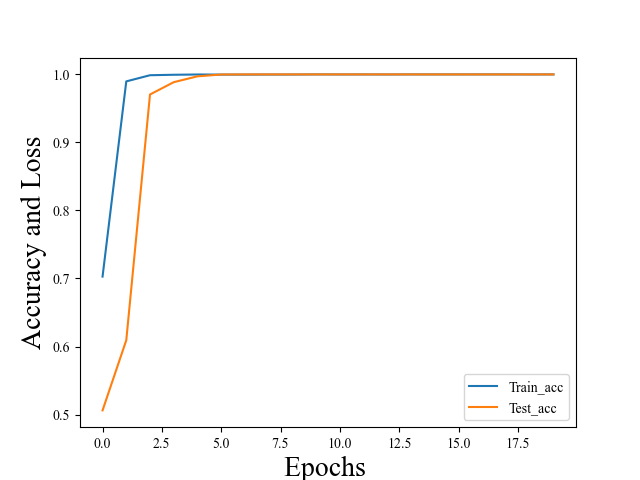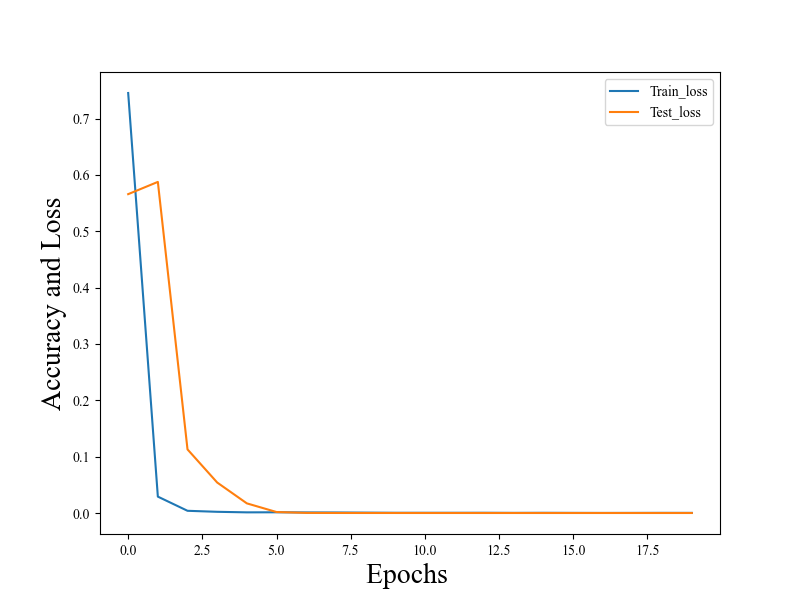我以轴承故障数据做了一个二分类，效果还可以，稍微些许可以调参解决。

展开全文人工智能 深度学习
• 维卷积神经网络，可以用来做一维的数据分析，以家用电器的识别分类作为背景。使用excel画出的简单的图形如下，横坐标为用电器开启后的秒数，纵坐标为某一秒的有功功率，由上至下分别为空调（Air Conditioner），...

维卷积神经网络，可以用来做一维的数据分析，以家用电器的识别分类作为背景。使用excel画出的简单的图形如下，横坐标为用电器开启后的秒数，纵坐标为某一秒的有功功率，由上至下分别为空调（Air Conditioner），冰箱（Refrigerator），烤炉（Stove）：
!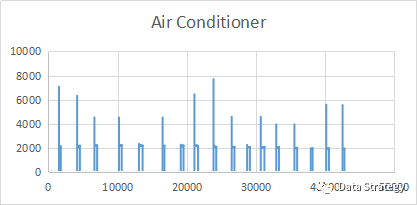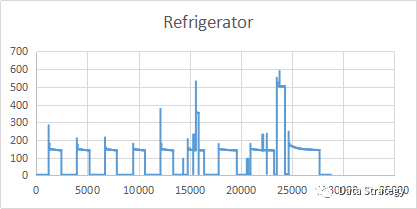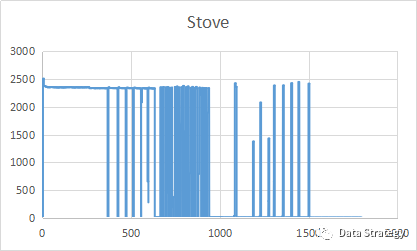从上面三个图可以看出不同的用电器在工作时会以自己特有的方式工作。从而形成不同的特征峰及平台。接下来使用到的数据一共有9类用电器，包括上面这三种，但是我这边的训练集仅有每分钟一个数据的训练集，如果有朋友有大量的一秒一个数据的dataset，可以分享一下哈，感恩。
一维卷积神经网络应用于电信号的分类的大致逻辑如下图：
!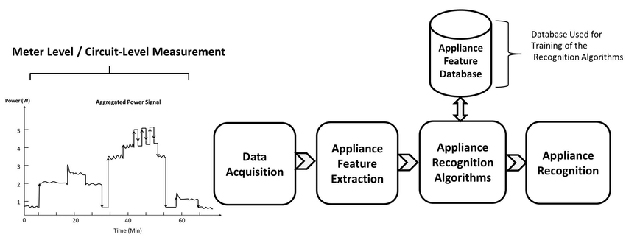对比于其它维度的卷积神经网络，其卷积运算过程（ConV)，池化过程(pooling)和全连接等可以理解为下图：
!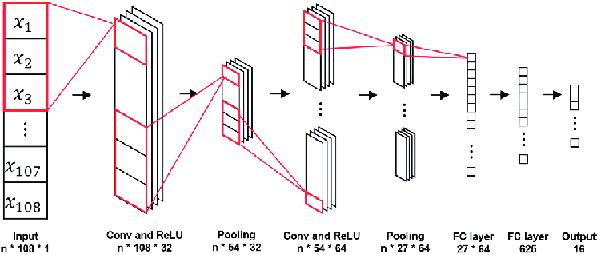具体的一维卷积运算过程如下图，蓝色框框就是我们的卷积核，也就是特征提取的detector，箭头方向即为我们卷积核的移动方向，因为是一维的，所以它只有一个方向上移动，而不像多维那样，往复：
!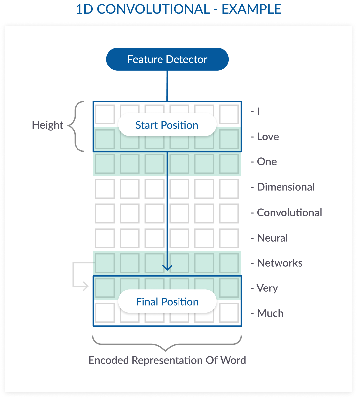一、导入包和数据：

import os
import csv
import keras
import pandas as pd
import numpy as np
from sklearn.metrics import classification_report
from sklearn.preprocessing import LabelEncoder,StandardScaler
from sklearn.model_selection import StratifiedShuffleSplit
from keras.models import Sequential
from keras.layers import Dense, Activation, Flatten, Convolution1D, Dropout
from keras.optimizers import SGD
from keras.utils import np_utils
import time
from datetime import datetime
from sklearn.datasets import make_blobs #generate isotropic Gaussian blobs for clustering
path=r'E:\ilm\train_data'
files=os.listdir(path)
column_names=[]
train=pd.DataFrame()
for item in files:
data_frame['mins']=range(len(data_frame))
data_frame=data_frame.drop(['Unnamed: 0'],axis=1)
data_frame=pd.pivot_table(data_frame,columns=['mins'])
train=train.append(data_frame)
train=train.reset_index()
train=train.rename(columns={'index':'types'})

!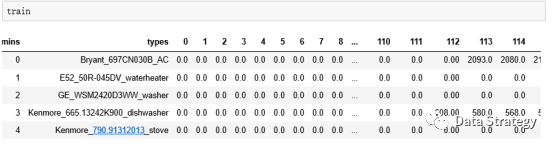从上图可以看到我们的表格分为两部分，一部分是label标签部分，变量名为types。另一部分是数值 value 部分，所用到的训练集均为两个小时的测量数据，一分钟一个数据，那么就一共有120个数据，从0到119一共120个变量。

二、LabelEncoder打标签
把数据导入进来，并处理好了之后，开始给他们编码label encoder()

label_encoder = LabelEncoder().fit(train.types)
labels = label_encoder.transform(train.types)
classes = list(label_encoder.classes_)
train = train.drop(['types'], axis=1)

LabelEncoder的用法
Encode target labels with value between 0 and n_classes-1。把标签贴上从0到n-1的数字, n为种类的数量。在这里为用电器的种类，在train的训练集里面，我们总共有9种用电器，所以这里label的code是0-8，一共9位。Labelencoder().fit(self,y) : fit label encoder, 将标签(types)和编码(code)一一对应起来。
transform(self,y): transform labels to normalized encoding. 把标签改成编码(code)。
其实上面fit和transform两部分可以用fit_transform function一步到位的。

三、零均值化和归一化
处理完label部分，接下来处理value部分。

scaler = StandardScaler().fit(train.values)
scaled_train = scaler.transform(train.values)

Standardscaler的用法sklearn.preprocessing.StandardScaler(copy=True,with_mean=True,with_std = True)
Standardize features by removing mean and scaling to unit variance. 这个过程就是上上一个章节讲到的zero-centered（零均值化）和normalization（归一化）的过程，如下图:
!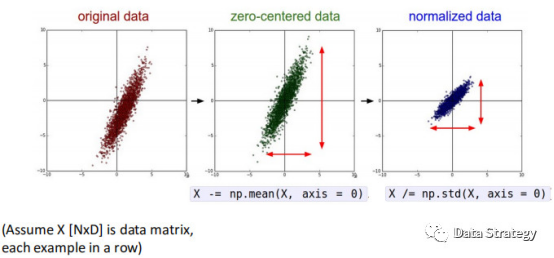StandardScaler().fit(self,X[,y]): compute the mean and std to be used for later scaling. 计算均值及标准差用以备用。

Transform(self,X[,copy]): perform standardization by centering and scaling. 零均值化和归一化使之标准化。这里的fit和transform这两部分也可以只用一个function来解决，使用fit_transform(self,X[,y])就行了。

四、把数据集随机拆分成训练/测试集
接下来把所有的数据分成train和test两部分，train部分用来训练模型，test部分用来检验模型的精确度。

sss = StratifiedShuffleSplit(n_splits=10,test_size=16, random_state=0) #
for train_index, valid_index in sss.split(scaled_train, labels):
#print("Train: ",train_index,"Test: ",valid_index)
X_train, X_valid = scaled_train[train_index], scaled_train[valid_index]
y_train, y_valid = labels[train_index], labels[valid_index]

StratifiedShuffleSplit的用法
sklearn.model_selection.StratifiedShuffleSplit(n_splits=10, test_size=None, train_size=None, random_state=None)
n_splits: Number of re-shuffling & splitting iterationstest_size（float, int, None, optional）：如果是介于0到1之间的浮点型，那么这个值就是用来做测试的数据量占总的数据量的一个百分比；如果是一个整数，那么这个值就是测试样品的数量；如果没有设置参数，那么测试的将会是所有数据；如果test_size这个参数没有设置，与此同时train_size也没有设置，那么这个值就会自动设置为0.1。
train_size（float, int, or None, default is None）: 顾名思义，其功能与test_size用法相似。
Random_state: If int, random_state is the seed used by the random number generator; if RandomState instance, random_state is the random number generator; if None, the random number generator is the RandomState instance used by np.random.
Random_state这个参数的设置与否决定了你产生的随机数的真假，当设置了这个参数时，产生的就是Pseudo-random number, 可以使你的结果重现，当没有设置这个参数时产生的就是真的随机数。下面我们把分别运行没有random_state这个参数两次和有这个参数两次，可以看到如下图的结果：
!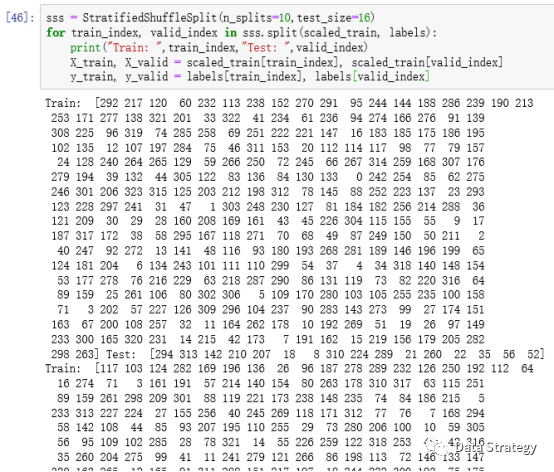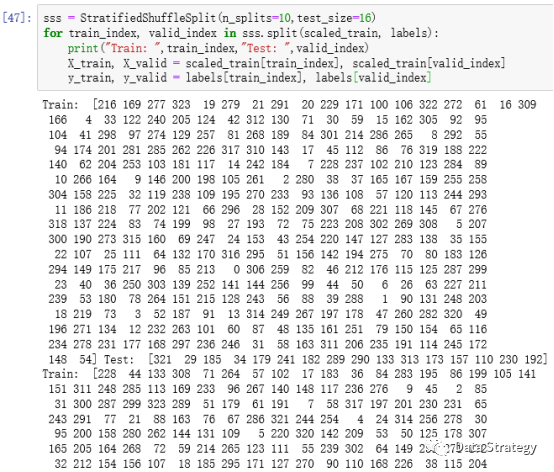上面两图中，相同的代码，所产生的随机数完全不一样，为真的随机数，完全随机将训练集和测试集分开。下面两个图中是加了参数的，分别运行两次后，两次的随机数是完全一模一样的，即为假的随机，所以在两次split中的training data set 和test data set的index是一样的（train dataframe中的每一行对应一个index）。
!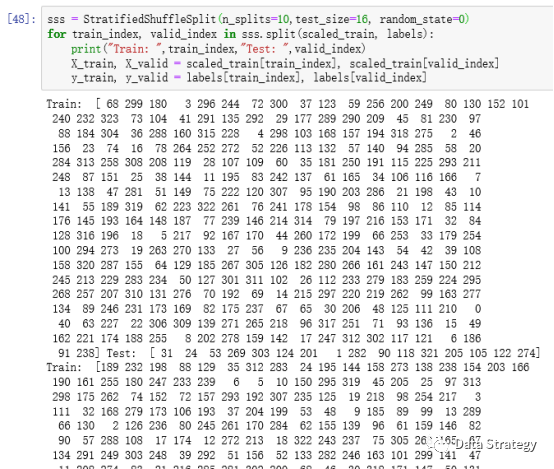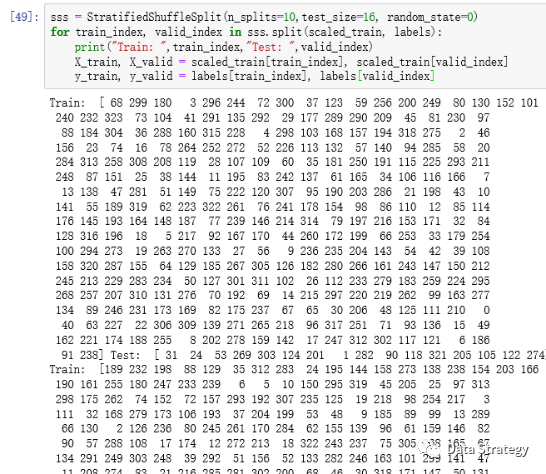上面就把train和test分好了，参数中设置的test_size=16，那么我们就用16个来test,其余的全部用来train, 通过print(train_index,valid_index) 列出来的index也可以看到我们用来test的data set里面有16组，这里我们一组就是一行，一行有一个label 和120个数据，y_train就是对应的用来train的那组数据的label，y_valid，就是用来test的那组数的label。

五、设置模型参数和reshape数据集
把训练/测试数据集分好后，接下来设置一些模型的参数和reshape数据集以符合模型需要：

nb_features = 120# number of features per features type (shape, texture, margin)
nb_class = len(classes) #nb_class=9

nb_features 每个特征类型的特征数，因为我这边是一维的，仅有一个功率的特征，长度都为120分钟，一分钟一个数据，一共两个小时，那么就是120个数据，所以这里我设置这个数值为120。对于有些多维的，如应用在图像识别里面，举个例子，给判别一片树叶的种类，树叶可以有不同的形状，纹理，边缘（锯齿），那么这就有三个特征，而每个特征如果用60个数值表示，那么这个number of features per features type就等于60。
而nb_class就是label的种类了，这边训练集中有9个种类，所以nb_class=9，或者直接读取之前Labelencoder时classes的长度。
为了符合keras中设定的维度，我们需要把我们的train data 进行reshape，所以这里我们先创建一个零矩阵，增加一个维度到3维（增加之前的train dataframe可以看作一个二维的数组，只有行和列），然后再用copy的方法，把数值复制进去。这里我们的电信号只有一个特征，所以第三个维度为1。如果是上面讲的树叶那个例子，因为判断树叶种类有三种特征（形状、纹理、边缘）那么第三个维度数值可以改为3，然后，X_train_r里面第三个维度的值就分别是0，1，2，每个所取得宽度都是60，因为每个特征有60个数值。在reshape validation data时跟reshape train data时情况是一样的。

# reshape train data
X_train_r = np.zeros((len(X_train), nb_features, 1))
X_train_r[:, :, 0] = X_train[:, :nb_features]
# reshape validation data
X_valid_r = np.zeros((len(X_valid), nb_features, 1))
X_valid_r[:, :, 0] = X_valid[:, :nb_features]

接着需要把标签矩阵转换成binary矩阵。

y_train = np_utils.to_categorical(y_train, nb_class)
y_valid = np_utils.to_categorical(y_valid, nb_class)

to_categorical的用法
to_categorical(y,num_classes=None,dtype=’float32’)
y: class vector to be converted into a matrix(integers from 0 to num_classes).
num_classes: total number of classes.
在这个分类模型中，因为之前编码的那0-8的数字，一共9位数，并没有代表着实际上的数学含义，而只是一个标签代表了该电信号所属的用电器种类，所以需要把该矩阵从class vector 转变为binary class 的matrix，最终输出的是一个 binary matrix。那么在这个例子中的具体情况就是一列变成了9列，里面的数值就是0或1，比如某一行属于这9个标签中的一个，那么对应的这行的矩阵在这个标签下的值就是1，其余值就是零，但是在其他的模型中有些情况是同时有多个标签的。个人觉得其实就是一个dummy variable，你觉得呢。如果只是二分类就不会有这么复杂了，如果不分类，那就是类似于数学中的线性拟合了。这个转变大致就是下图这样的过程。
!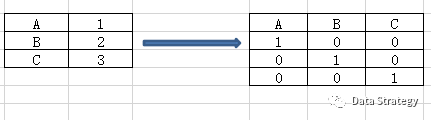数据和参数差不多已经设置完毕，下面开始建立模型了。

六、建立模型

# Keras model with one Convolution1D layer
# unfortunately more number of covnolutional layers, filters and filters length don't give better accuracy
model = Sequential()model.add(Convolution1D(nb_filter=4, filter_length=3, input_shape=(nb_features, 1)))
# nb_filter:Number of convolution kernels to use(dimensionality of the output)
# filter_length: The extension (spatial or temporal) of each filter.model.add(Activation('relu'))
model.add(Activation('softmax'))

Sequential API是建立keras models的方法之一，简单方便，可以一层层地建模，但是不能分享层或者有多元地输入或者输出。另外，functional API 可以允许你创建更灵活的模型，可以使层链接上一层或下一层，事实上也可以链接到任何一层，正因为如此，functional API 可以可以创建复杂的网络，例如siamese networks 和residual networks。Siamese networks 是一种孪生神经网络，可以衡量两个输入的相似程度，即可以有多个输入。这里就不再拓展了，有兴趣的可以自己看一下。
number of filter设置的数量越多，在model.fit的时候运算量就越大，但是运算量越大，不一定你的模型就越精确，比如我刚开始设置的值是512，后来还试过12和4，发现12和4他们测试的准确性都差不多，所以就没有必要设到512，具体还是需要多测试一些参数，然后找到合适的。

sgd = SGD(lr=0.01, nesterov=True, decay=1e-6, momentum=0.9)
model.compile(loss='categorical_crossentropy',optimizer=sgd,metrics=['accuracy'])nb_epoch = 50
model.fit(X_train_r, y_train, nb_epoch=nb_epoch, validation_data=(X_valid_r, y_valid))# 我把, batch_size=32删掉了
# 往返（顺逆）一次一个epoch，每次都做一个split，分成两部分，一部分用来training(training data),
# 另一部分用来评估（validation data),
# it trains the model on training data and validate the model on validation data by checking its loss and accuracy.

sgd = SGD(lr=0.01, nesterov=True, decay=1e-6, momentum=0.9)
Lr: learning rate, float>=0.
学习速率控制模型对于预测值和实际值的差值的调整速度，过小会导致长时间的训练，小的学习速率learning rate需要更多的训练次数(training epoch), 大的学习速率因为更快的调整改变速度，所需要的training epoch就会更少，那么对应设置的epoch参数也会更小；学习速率太快会引起模型过快收敛，过小则会造成stuck。
Nesterov: boolean, whether to apply nesterov momentum.
Momentum: float>=0. Parameter that accelerates SGD in the relevant direction and dampens oscillations. 在某些方向上加速梯度下降，并且对震动产生阻尼。
Momentum 和 Nesterov Momenturm 可以加速训练并且提升收敛度，同时momentum 还可以避免跌入到局部最小。decay: decay rate which is greater than zero and iteration is the current update number. 这个decay是应用在learning rate这个参数上的，就是将学习速率衰减，因为在最开始的时候我们需要较高的学习速率，而在接近global minimum(or maximum)的时候需要的学习速率较小，所以就存在一个learning rate变化的过程，那么调节这个变化的参数我们用decay（衰减系数）来设置，learning rate decay也有很多种方式，比如分段常数衰减，反时限衰减和多项式衰减等。
与SGD相关的数学问题，可以参阅下面的链接：
https://dominikschmidt.xyz/nesterov-momentum/
compile(optimizer, loss=None, metrics=None, loss_weights=None, sample_weight_mode=None, weighted_metrics=None, target_tensors=None)
compile: configures the model for training.
optimizer: 最小化和最大化目标函数，中文有人叫优化器。loss: loss function. 损失函数。
这篇文章举的例子是一个多分类问题，所以用categorical_crossentropy, binary_crossentropy 为二分类交叉熵损失，softmax 使用的即为交叉熵损失函数。
nb_epoch: number of epoch,一个来回叫一个epoch，这里把模型训练50次的意思，训练过多会出现过拟合，过少可能欠拟合。不过也可以用early stopping 来防止过拟合，callbacks 中的 EarlyStopping 是在每一个epoch结束时，计算validation data的accuracy, 当accuracy 不再提高时就停止训练。
Model.fit(x=None,y=None,batch_size=None,epochs=1,callbacks=None,validation_data=None…)Model.fit() 就是拟合过程，其他参数上面已经解释过了，那么我们来看看batch_size。
Batch_size: number of samples per gradient update. 自定义的是32，如果你用的是symbolic tensors, generators 或者 sequence instance，那么就不需要设置这个bathes_size 参数，因为他们会自己产生，所以我这里没有设置batch_size的值。

七、做分类预测下面用训练好的模型做一个预测：

#predict
compare_data['mins']=range(len(compare_data))
test=pd.pivot_table(compare_data,columns=['mins'])
test_r = np.zeros((len(test), 120, 1))
test_r[:, :, 0] = test.values[:, :120]
# there are two types of classification predictions: class predictions and probability predictions.
test_y=model.predict_classes(test_r)
test_y_prob=model.predict_proba(test_r)

predict_classes(self, x, batch_size=32, verbose=1)对输入的数据进行分类判断。得到的test_y结果如下。
!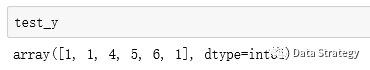predict_proba(self,X) 返回的是一个类似于刚刚binary matrix的矩阵。
!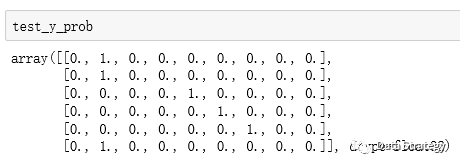因为之前测试的时候也是一组只有一个标签，那么在这个标签下的概率是完整的1，如果它同时有多个标签，那么就会有多个标签分别对应的一个概率，那么得到的矩阵也就不是非0即1了，如果是多个标签那种情况的话，那还需要在整行中寻找概率最大的那个值对应的标签。也可以用下面这种方式来处理：

label_encoder.inverse_transform(test_y)

inverse_transform(self,y) Transform labels back to original encoding. 将encoded之后的数值转化为原来的类型名字。然后把test_y的预测分类结果所对应的types找出来了。
!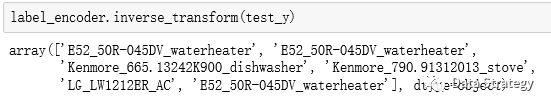!

扫描下方二维码，或者搜索微信公众号： DataStrategy 关注微信。
后台回复“一维卷积神经网络应用于电信号分类”（建议复制）即可以获得训练数据集的压缩包了。展开全文机器学习 人工智能 python
• 提出种基于深度体素卷积神经网络的三(3D)模型识别分类算法, 该算法使用体素化技术将3D多边形网格模型转化为体素矩阵, 并通过深度体素卷积神经网络提取该矩阵的深层特征, 以增强特征的表达能力和差异性。...
• 结合高光谱影像中的空间特征与光谱信息，提出种适合于高光谱像素级分类的深度学习三维卷积神经网络模型(3D-CNN)，并在初始分类的基础上利用多标签条件随机场进行优化。选取三个通用公开高光谱数据集(Indian Pines...
• 卷积神经网络 卷积神经网络（ConvolutionalNeuralNetwork，CNN）最初是为解决图像识别等问题设计的，CNN现在的应用已经不限于图像和视频，也可用于时间序列信号，比如音频信号和文本数据等。CNN作为个深度学习...
• 采用一维CNN神经网络算法，对西储大学轴承数据集分为10中故障类型进行故障识别，最终准确率很高；同时算法结构灵活，可以自定制网络及优化器，满足多张故障数据集。
• 针对传统计算机辅助检测系统中肺结节检测存在大量假阳性的问题,提出种基于三维卷积神经网络的肺结节识别方法。首先,将传统二维卷积神经网络扩展为三维卷积神经网络,充分挖掘肺结节的三维特征,增强特征的表达能力;...
• 卷积神经网络一般用来处理图像信息，对于序列这种一维的数据而言，我们就得采用一维的卷积，tensorflow中提供有专用的函数conv1d，各参数的使用说明如下： conv1d参数 说明 value 输入数据，value的格式为：...
• 要对输出进行分类，请包括个输出大小为 K 的全连接层、个 softmax 层和分类层，其中 K 是类的数量。 首先，在个层数组中，指定输入层、首个一元分词块、深度串联层、全连接层、softmax 层和分类层。 ...
• 针对人工提取高分辨率距离像（High Range Resolution Profile, HRRP）优良特征比较困难的问题，研究了基于一维卷积神经网络（Convolutional Neural Network, CNN）的HRRP识别方法。利用CNN具有分层学习特征的能力，...
• CNN卷积神经网络Matlab实现例程，使用了matlabR2019a自带的深度学习工具箱,一维数据分类，二维cnnmatlab
• (164条消息) 一维卷积神经网络_卷积神经网络的基础知识_weixin_39526651的博客-CSDN博客 这篇博客给的图文解释非常棒，欢迎学习 (165条消息) 一维卷积_perfect_csdn1的博客-CSDN博客_一维卷积 参考资料 ...1dcnn
• 下面我所写的是卷积神经网络中的一维卷积，其整体思想差不多。 卷积的定义 卷积(Convolution)，也叫做褶积，是分析数学的一种重要的运算，在信号处理和图像处理中，经常使用一维或者二位卷积。 一维卷积 一维卷积...深度学习
• 本帖最后由 yexu55066 于 2016-8-16 02:17 编辑我用的DeepLearnToolbox的代码改了改成一维卷积神经网络，来识别一维的心电信号，训练样本40000均分成三类，训练后发现训练部分错误率就超高，到测试集就把所有信号都...
• 本文为挪威奥斯陆大学（作者：Sharanan Kulam）的硕士论文，共176页。 近年来，机器智能的研究取得了很大的进展，...因此，近年来卷积神经网络（CNN）被广泛应用于序列建模，其性能优于RNN。然而，这种效率仅通过少数.
• 深度学习之卷积神经网络 基本的图像分类模型架构 卷积层：用来提取图像的底层特征 池化层：防止过拟合，减小数据维度 全连接层：汇总卷积层和池化层得到的底层特征和信息，再进行输出。 使用padding：为了防止边缘...深度学习
• 基于特征融合一维卷积神经网络的电能质量扰动分类.pdf
• 基于一维卷积神经网络的化爆和地震次声分类.pdf
•研究论文
• 基于一维卷积神经网络的雌激素粉末拉曼光谱定性分类.pdf
• 基于一维卷积神经网络的患者特异性心拍分类方法研究.pdf
• 文章目录使用智能手机数据集进行活动识别开发一维卷积神经网络拟合和评估模型总结结果调整一维卷积神经网络过滤器数量内核大小多头卷积神经网络一些拓展的想法 人类活动识别是将专用线束或智能手机记录的加速度计...机器学习 人工智能 目标识别 opencv
• 本文以MNIST手写数字分类为例，讲解使用一维卷积和二维卷积实现 CNN 模型。关于 MNIST 数据集的说明，见使用TensorFlow实现MNIST数据集分类。实验中主要用到 Conv1D 层、Conv2D 层、MaxPooling1D 层和 MaxPooling2D...
• MATLAB实现一维卷积神经网络 Crack Identification From Accelerometer Datamatlab GRU 1D-CNN
• 基于一维卷积神经网络的滚动轴承故障识别 提示：文章写完后，目录可以自动生成，如何生成可参考右边的帮助文档 文章目录基于一维卷积神经网络的滚动轴承故障识别一、数据预处理二、模型搭建三、使用步骤1.引入库2....深度学习
• 为了进一步提高点云图像船舶分类方法的分类准确率,提出了种基于三维卷积神经网络(3D CNN)的点云图像船舶分类方法。首先采用密度网格方法将点云图像转为体素网格图像,将体素网格图像作为3D CNN的输入对象;接着通过......